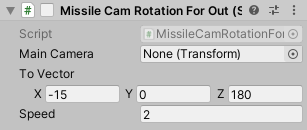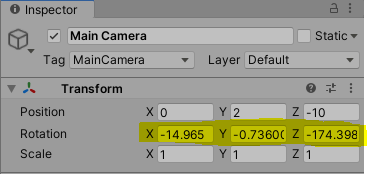# A Problem With Quaternion.Slerp

I wrote a code block to rotate the main camera. However, the result is not what I want.

public Transform mainCamera;

``````    public Vector3 toVector;

public float speed;
private float timeCount = 0.0f;

private Quaternion from;
private Quaternion to;

private void Awake()
{
mainCamera = GameObject.FindGameObjectWithTag("MainCamera").transform;
}

private void OnEnable()
{
from = mainCamera.localRotation;
to = Quaternion.Euler(toVector);
}

void Update()
{
mainCamera.localRotation = Quaternion.Slerp(from, to, timeCount);
timeCount = timeCount + speed * Time.deltaTime;
if (timeCount > 1.0f)
{
timeCount = 0.0f;
this.enabled = false;
}
}
``````Result;How to fix it?

I modified your script a little. Seems to work fine now. You might want to consider using WaitForSeconds (Unity - Scripting API: WaitForSeconds) instead but for now this seems to work fine. Hope this helps.

``````    public Transform mainCamera;

public Vector3 toVector;

public float speed;
private float timeCount = 0.0f;

private Quaternion from;
private Quaternion to;

private bool rotating;

private void Awake()
{
mainCamera = GameObject.FindGameObjectWithTag("MainCamera").transform;
rotating = true;
}

private void OnEnable()
{
from = mainCamera.localRotation;
to = Quaternion.Euler(toVector);
}

void Update()
{
if (rotating)
{
mainCamera.localRotation = Quaternion.Slerp(from, to, timeCount);
timeCount = timeCount + speed * Time.deltaTime;
if (timeCount >= 1.0f)
{
rotating = false;
mainCamera.localRotation = to;
}
}
}
``````

It works… Perfect.
Thank you so much…